# Questions with Answers: Speed, Time & Distance- 1 Notes | Study Quantitative Aptitude (Quant) - CAT

## CAT: Questions with Answers: Speed, Time & Distance- 1 Notes | Study Quantitative Aptitude (Quant) - CAT

The document Questions with Answers: Speed, Time & Distance- 1 Notes | Study Quantitative Aptitude (Quant) - CAT is a part of the CAT Course Quantitative Aptitude (Quant).
All you need of CAT at this link: CAT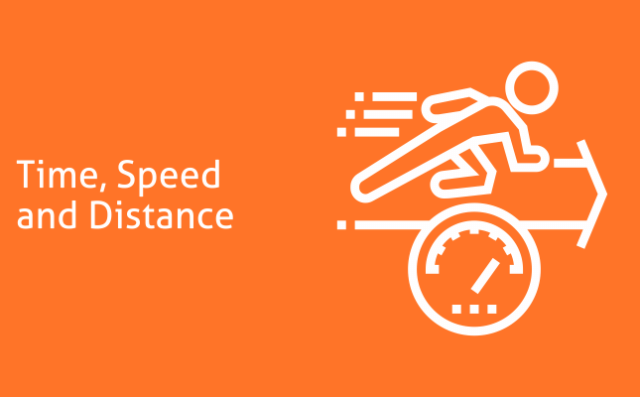Question 1: Two friends A and B simultaneously start running around a circular track. They run in the same direction. A travels at 6 m/s and B runs at b m/s. If they cross each other at exactly two points on the circular track and b is a natural number less than 30, how many values can b take?
(A) 3
(B) 4
(C) 7
(D) 5

• Let track length be equal to T.
• Time taken to meet for the first time =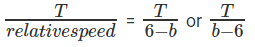• Time taken for a lap for A = T/6
• Time taken for a lap for B = T/b
• So, time taken to meet for the first time at the starting point = LCM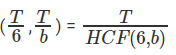• Number of meeting points on the track = Time taken to meet at starting point/Time taken for first meeting = Relative speed / HCF (6,b).
• So, in essence we have to find values for b such that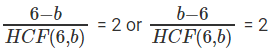• The question is " If two people cross each other at exactly two points on the circular track and b is a natural number less than 30, how many values can b take?"
• b = 2, 10, 18 satisfy this equation. So, there are three different values that b can take.

Question 2: Consider a square ABCD. EFGH is another square obtained by joining the midpoints of the sides of the square ABCD where E, F , G amd H are the midpoints of AB, BC, CD and DA respectively. Lakshman and Kanika start from points B and D respectively at speeds ‘l’ kmph and ‘k’ kmph respectively and travel towards each other along the sides of the square ABCD. Jagadeesh starts from Point E and travels along the Square EFGH in the anti-clockwise direction at ‘j’ kmph. Lakshman and Kanika meet for the second time at H where Jagadeesh also meets them for the first time. If l : k : j is 1: 3 : 5√2 then the distance travelled by Jagadeesh is:
(A) 7.5 × √2 times the side of the square ABCD
(B) 7.5 × √2 times the side of the square EFGH
(C) 7.5 times the side of the square ABCD
(D) 7.5 times the side of the square EFGH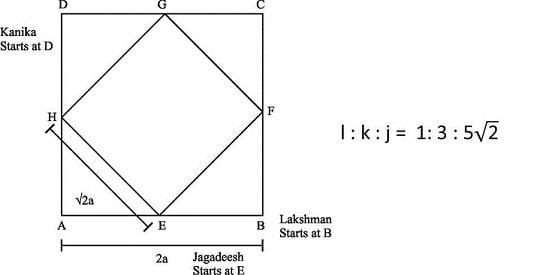• Lakshman and Kanika start from points B and D respectively at speeds ‘l’ kmph and ‘k’ kmph respectively and travel towards each other along the sides of the square ABCD. They are at a distance of 4a from each other.
• Since they are at diametrically opposite points, the relative distance would be 4a irrespective of the directions they choose to travel in. So, to meet for the first time, they would have travelled a distance of 4a together.
• To meet for the second time, they would have travelled a further 8a together. Essentially, between them, they would have to cover the entire perimeter of the square to meet again.
• So by the time they meet for the second time, they would have covered a distance of 12a together. Their speeds are in the ratio 1: 3. So, Lakshman would have travelled 3a and Kanika would have travelled 9a.
• Or, Lakshman travels in the direction BADC, while Kanika would have travelled in the direction DABC. They meet for the first time at E and the second time at H.
In the same time, Jagadeesh travels along the square EFGH in the anti-clockwise direction at ‘j’ kmph and meets Lakshman and Kanika.
• While Jagadeesh meets the other two for the first time, we do not know how many laps he has completed by then.
• The ratio of Lakshman’s speed to that of Jagadeesh is 1: 5√2 so, they would have travelled distances in the same ratio as well. So, if Lakshman has travelled a distance of 3a, Jagadeesh should have travelled a distance of 3a x 5√2 to reach H.
• The question is "If l : k : j is 1: 3 : 5√2 then the distance travelled by Jagadeesh is"
Hence, the answer 7.5 × √2 times the side of the square ABCD.

Question 3: Three cars leave A for B in equal time intervals. They reach B simultaneously and then leave for Point C which is 240 km away from B. The first car arrives at C an hour after the second car. The third car, having reached C, immediately turns back and heads towards B. The first and the third car meet a point that is 80 km away from C. What is the difference between the speed of the first and the third car?
(A) 60 kmph
(B) 20 kmph
(C) 40 kmph
(D) 80 kmph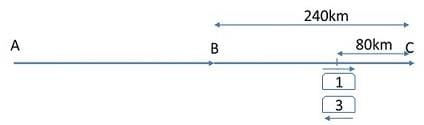• Let V1, V2 and V3 be the speeds of the cars.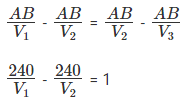• V3 = 2V1
• Condition I states that the cars leave in equal intervals of time and arrive at the same time. Or, the difference in the time taken between cars 1 and 2 should be equal to the time taken between cars 2 and 3.
• We get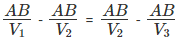• As the second car arrived at C an hour earlier than the first, we get a second equation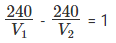• The third car covered 240 + 80 kms when the first one covered 240 – 80 kms.
• Therefore,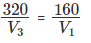This gives us V3 = 2V1
• From condition 1, we have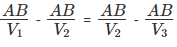• Substituting V3 = 2V1, this gives us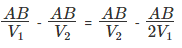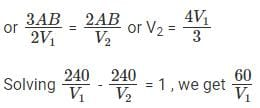= 1 or V1 = 60 kmph
=> V2 = 80 kmph and V3 = 120 kmph
• The question is "What is the difference between the speed of the first and the third car?"
Hence, the answer is 60 kmph.

Question 4: Three friends A, B and C decide to run around a circular track. They start at the same time and run in the same direction. A is the quickest and when A finishes a lap, it is seen that C is as much behind B as B is behind A. When A completes 3 laps, C is the exact same position on the circular track as B was when A finished 1 lap. Find the ratio of the speeds of A, B and C?
(A) 5 : 4 : 2
(B) 4 : 3 : 2
(C) 5 : 4 : 3
(D) 3 : 2 : 1

• Let track length be equal to T. When A completes a lap, let us assume B has run a distance of (t - d). At this time, C should have run a distance of (t - 2d).
• After 3 laps C is in the same position as B was at the end of one lap. So, the position after 3t - 6d should be the same as t - d. Or, C should be at a distance of d from the end of the lap.
• C will have completed less than 3 laps (as he is slower than A), so he could have traveled a distance of either t - d or 2t - d.
=> 3t - 6d = t - d
=> 2t = 5d
=> d = 0.4t
• The distances covered by A, B and C when A completes a lap will be t, 0.6t and 0.2t respectively. Or, the ratio of their speeds is 5 : 3 : 1.
• In the second scenario, 3t - 6d = 2t - d => t = 5d => d = 0.2t.
The distances covered by A, B and C when A completes a lap will be t, 0.8t and 0.6t respectively. Or, the ratio of their speeds is 5 : 4 : 3.
• The question is " Find the ratio of the speeds of A, B and C?"
The ratio of the speeds of A, B and C is either 5 : 3 : 1 or 5 : 4 : 3.
• Hence, the answer is 5 : 4 : 3

Question 5: Mr. X decides to travel from Delhi to Gurgaon at a uniform speed and decides to reach Gurgaon after T hr. After 30 km, there is some engine malfunction and the speed of the car becomes 4/5th of the original speed. So, he travels the rest of the distance at a constant speed 4/5th of the original speed and reaches Gurgaon 45 minutes late. Had the same thing happened after he travelled 48 km, he would have reached only 36 minutes late. What is the distance between Delhi and Gurgaon?
(A) 90 km
(B) 120 km
(C) 20 km
(D) 40 km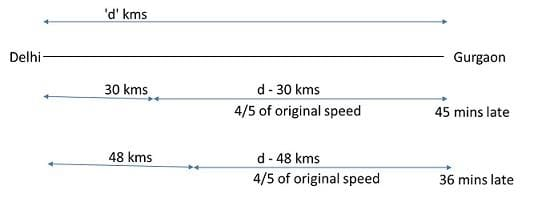• Let the distance from Delhi to Gurgaon be ‘d’ km.
• The first 30 km he travels at his usual speed. However, the remaining ‘d-30’ km he travels at a reduced speed.
• To travel ‘d’ km he usually takes T hr. Therefore, to travel ‘d - 30’ km he should ideally take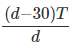hr.
• However, this is only if he travels at his usual speed. It is given that he traveled only at 4/5th of his usual speed. Because of this he would have taken 5/4th of the time to travel the remaining distance, i.e., he takes  1/4th of the time extra. This is given to be 45 minutes (or 3/4th hr)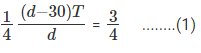• On the other hand, had the same thing happened after he travelled 48 km, he would have reached only 36 minutes or 34 hrs late. Hence,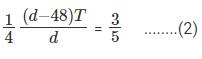• Dividing (1) by (2) and solving for d, we get d = 120 km.
• The question is What is the distance between Delhi and Gurgaon? we get d = 120 km.
• Hence, the answer is d = 120 km.

Question 6: Two friends A and B leave City P and City Q simultaneously and travel towards Q and P at constant speeds. They meet at a point in between the two cities and then proceed to their respective destinations in 54 minutes and 24 minutes respectively. How long did B take to cover the entire journey between City Q and City P?
(A) 60
(B) 36
(C) 24
(D) 48

• Let us assume Car A travels at a speed of a and Car B travels at a speed of b.
Further, let us assume that they meet after t minutes.
• Distance traveled by car A before meeting car B = a * t. Likewise distance traveled by car B before meeting car A = b * t.
• Distance traveled by car A after meeting car B = a * 54. Distance traveled by car B after meeting car A = 24 * b.
• Distance traveled by car A after crossing car B = distance traveled by car B before crossing car A (and vice versa).
=> at = 54b ---------- (1)
and bt = 24a -------- (2)
• Multiplying equations 1 and 2
we have ab * t2 = 54 * 24 * ab
=> t2 = 54 * 24
=> t = 36
• The question is " How long did B take to cover the entire journey between City Q and City P?"
• So, both cars would have traveled 36 minutes prior to crossing each other. Or, B would have taken 36 + 24 = 60 minutes to travel the whole distance.
• Hence, the answer is 60 mins.

Question 7: A swimming pool is of length 50 m. A and B enter a 300 m race starting simultaneously at one end of the pool at speeds of 3 m/s and 5 m/s. How many times will they meet while travelling in opposite directions before B completes the race?
(A) Twice
(B) Thrice
(C) Once
(D) 5 Times

• Let us say the pool starts from P and ends at Q. B being the quicker one will reach Q first. B will reach Q after 10 seconds. A will take 50/3 = 16.67 seconds to reach Q, so between 10s and 16.67 seconds they meet once.
• First, let us have a table where we know times when A, B would be at the end points.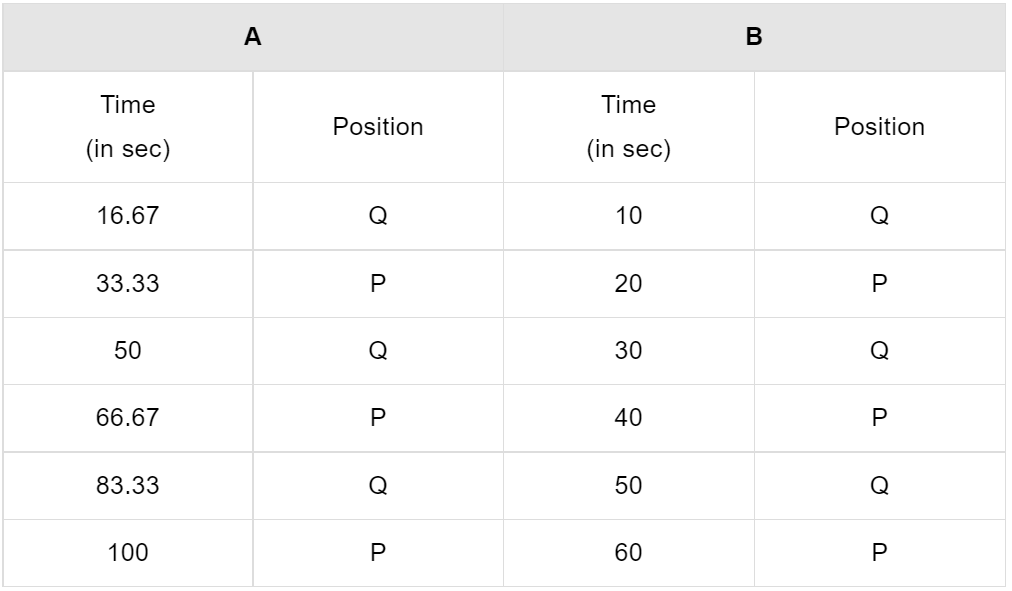• We see that the critical time slots are multiples of 10 seconds and multiples of 16.67 seconds.
• So, let us break the time from 0 to 60 seconds into these slots and see in which direction A and B travel.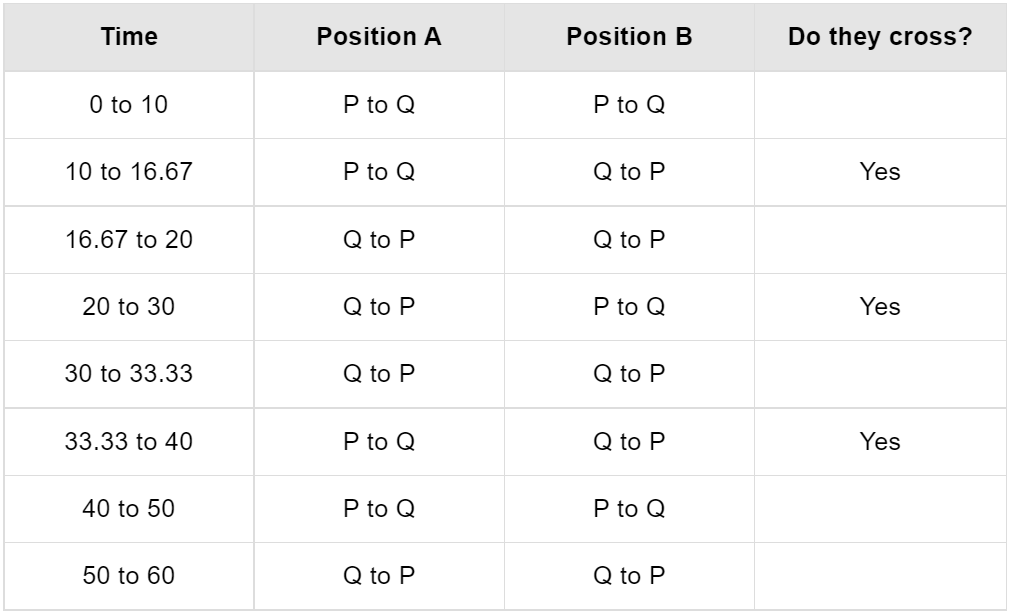• So, the question is, how many times will they meet before B finishes the race?
They cross each other thrice totally. Between 10 and 16.67 seconds for the first time; between 20 and 30 seconds for the second time; and between 33.33 to 40 seconds for the third time.
• Hence, they meet 3 times before B finishes the race.
The document Questions with Answers: Speed, Time & Distance- 1 Notes | Study Quantitative Aptitude (Quant) - CAT is a part of the CAT Course Quantitative Aptitude (Quant).
All you need of CAT at this link: CATUse Code STAYHOME200 and get INR 200 additional OFF

## Quantitative Aptitude (Quant)

167 videos|152 docs|131 tests

### How to Prepare for CAT

Read our guide to prepare for CAT which is created by Toppers & the best Teachers

Track your progress, build streaks, highlight & save important lessons and more!

,

,

,

,

,

,

,

,

,

,

,

,

,

,

,

,

,

,

,

,

,

,

,

,

;